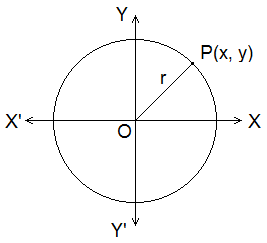# Equation of a Circle## Equation of a Circle

Equation of a circle is a second degree equation in x and y which satisfies all the points on the circumference of a circle in a Cartesian Plane. If r is the length of radius and (a, b) is the centre of a circle, then this circle has an equation (x – a)2 + (y – b)2 = r2

**********************

10 Math Problems officially announces the release of Quick Math Solver, an android APP on the Google Play Store for students around the world.**********************

An equation of the form x2 + y2 + 2gx + 2fy + c = 0 represents a circle if g2 + f2 – c > 0, and is then an equation of the circle with centre at (–g, –f) and radius √(g2 + f2 – c).

###Equation of a Circle With Centre at Origin

Let O(0, 0) be the centre of a circle. If P(x, y) be any point on the circumference of the circle, then OP = r.
By using distance formula,
OP2 = (x – 0)2 + (y – 0)2
x2 + y2 = r2           … … … (i)
The relation (i) is satisfied by every point on the circle, hence it represents the equation of circle with centre at origin and radius r.

Example 1: Find the equation of circle with centre at origin and radius 5 units.
Solution:
Here, radius (r) = 5 units
The equation of a circle with centre at origin is
x2 + y2 = r2
or,          x2 + y2 = 52
x2 + y2 = 25 is the required equation.

### Equation of Circle With Centre at (h, k)

By using distance formula,
CP2 = (x – h)2 + (y – k)2
or,          (x – h)2 + (y – k)2 = r2      … … … (i)
This relation is true for every point on the circle. Hence, represents a circle with centre at (h, k) and radius r.
Equation (i) can be written as
x2–2hx+h2+y2–2ky+k2–r2 = 0
or,          x2+y2–2hx–2ky+h2+k2–r2 = 0
or,          x2 + y2 + 2gx + 2fy + c = 0               … … … (ii)
where g = -h, f = -k and c = h2 + k2 – r2.
The equation (ii) is said to be the General equation of circle. This equation of circle has the following characteristics.
a.       It is second degree in x and y.
b.      Coefficient of x2 = coefficient of y2
c.       Coefficient of xy = 0.
Thus the general equation of second degree ax2 + 2hxy + by2 + 2gx + 2fy + c = 0 will represent a circle if a = b and h = 0.

Example 2: Find the equation of a circle whose centre is at point (-4, 3) and radius 4 units.
Solution:
Here, centre of circle is (-4, 3)
i.e. h = -4, k = 3 and radius (r) = 4.
The equation of circle with centre:(h, k) and radius=r is
(x–h)2+(y – k)2= r2
or,          (x + 4)2 + (y – 3)2 = 42
or,          x2 + 8x +16 + y2 – 6y + 9 = 16
x2 + y2 + 8x – 6y + 9 = 0 is the required equation.

Example 3: Find the centre and radius of the circle x2 + y2 – 6x + 4y – 23 = 0
Solution:
The given equation is
x2+y2–6x+4y–23 = 0
or,          x2 – 6x + y2 + 4y – 23 = 0
or,          x2 – 2.x.3 + 32 + y2 + 2.y.2 + 22 = 23 + 32 + 22
or,          (x – 3)2 + (y + 2)2 = 23 + 9 + 4
or,          (x – 3)2 + (y + 2)2 = 62
Comparing it with (x – h)2 + (y – k)2 = r2, we have h = 3, k = -2 and r = 6
Hence, centre of circle is (h, k) = (3, - 2) and radius r = 6.

### Equation of Circle in a Diameter FormLet O be the centre and A(x1, y1) and B(x2, y2) be the ends of diameter AB of the circle. If P(x, y) be any point on the circumference of the circle, then

Since, APB is the angle in the semi-circle APB = 90°.
m1 × m2 = -1

or,          (y – y1)(y – y2) = - (x –x1)(x – x2)
or,          (x – x1)(x – x2) + (y – y1)(y – y2) = 0
Hence, it is the equation of circle in diameter form.

Example 4: Find the equation of a circle whose ends of the diameter are at (-1, 3) and (5, 7).
Solution:
Here, ends of the diameter of the circle are (-1, 3) and (5, 7).
i.e. x1 = -1, y1 = 3, x2 = 5 and y2 = 7.
The equation of circle in diameter form is
(x–x1)(x–x2) + (y–y1)(y–y2) = 0
or,          (x + 1)(x – 5) + (y – 3)(y – 7) = 0
or,          x2 – 5x + x – 5 + y2 7y – 3y + 21 = 0
or,          x2 + y2 – 4x – 10y + 16 = 0 is the required equation.

Example 5: Find the equation of a circle with centre (-3, 6) and touching the x-axis.
Solution:Here, centre of circle is (-3, 6). As the circle touches the x-axis, radius of the circle is 6.
Then the equation of the circle is
(x–h)2 + (y–k)2 = r2
or,          (x + 3)2 + (y – 6)2 = 62
or,          x2 + 6x + 9 + y2 – 12y + 36 = 36
x2 +y2 +6x -12y + 9 = 0 is the required equation or circle.

You can comment your questions or problems regarding the equation of circle here.